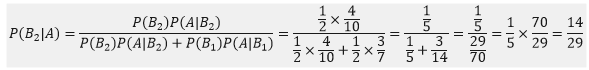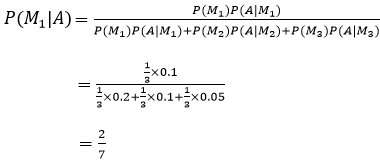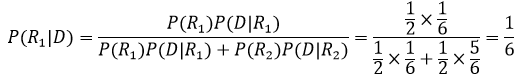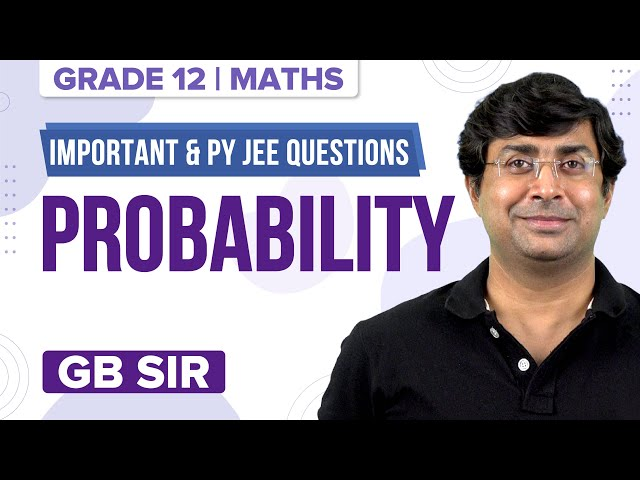Win up to 100% scholarship on Aakash BYJU'S JEE/NEET courses with ABNAT Win up to 100% scholarship on Aakash BYJU'S JEE/NEET courses with ABNAT

# Bayes Theorem of Probability

## Bayes’ Theorem Introduction

Bayes’ Theorem governs the likelihood that one event is based on the occurrence of some other events. It depends upon the concepts of conditional probability. This theorem gives us the probability of some events depending on some conditions related to the event.

We know that the likelihood of heart disease increases with increasing age. So, if we know someone’s age and know the chances of getting heart disease, we can easily find the possibility of the person having a heart disease.

Let us take another example. We have been given a bag of green, white and red balls. It’s been said that we have to pick a green ball only after picking a blue ball. It’s been sure that we can only pick green balls after picking a blue ball. This makes the case of conditional probability.

## The Formula of Bayes’ Theorem

$$\begin{array}{l}P(A|B) = \frac{P(A).P(B|A)}{P(B)}\end{array}$$

Where:

P(A) = Probability of occurrence of event A

P(B) = Probability of occurrence of event B

P(A|B) = Probability of occurrence of event A given B

P(B|A) = Probability of occurrence of event B given A

## Bayes’ Theorem Statement

Let B1, B2, B3, ….., Bn be a series of n non-empty sets with sample space as X, where all the sets have a non-zero probability of occurrence, and they form a partition of X. Let E be an event associated with X, then by Bayes’ Theorem, we get:

$$\begin{array}{l}P(B_i|X) = \frac{P(B_i)P(X|B_i)}{\sum_{j=1}^n P(B_j)P(A|B_j)}\end{array}$$

For all i = 1, 2, 3, ……,n

### Bayes’ Theorem Derivation

According to conditional probability, we get,

$$\begin{array}{l}P(B_i|X) = \frac{P(B_i)P(X|B_i)}{P(A)}\end{array}$$
[By multiplicative property]
$$\begin{array}{l}=\frac{P(B_i)P(X|B_i)}{\sum_{j=1}^n P(B_j)P(X|B_j)}\end{array}$$
[By total probability theorem]

Each of these occurrences B1, B2, B3, ….., Bn is called a hypothesis.

The probability P(Bi) is known as a prior probability of the hypothesis Bi.

The probability of P(Bi|X) is called a posteriori probability of the hypothesis Bi.

The significance of Baye’s theorem can be understood in the following ways.

The experiment can be performed in n mutually exclusive and exhaustive ways B1, B2…Bn. The probability P(Bi) of the occurrence of event Bi, i = 1, 2, ..n is known. The experiment is done, and we know that event X has occurred. With this information, the probability P(Bi) is changed to P(Bi/X). This theorem enables us to evaluate P(Bi/X) if all the P(Bi) priori probabilities and P(X/Bi) likelihood probabilities are known.

### Bayes’ Theorem Problems

Example 1: Consider 2 bags. Bag 1 is made full by putting three red and four blue balls. Bag 2 is made full by putting four red and six blue balls. We need to find out the value of the probability of drawing a red ball from the second bag. So, we need to find out from which bag the red ball will be drawn.

Solution: Let us take B1 be the chance of choosing Bag 1,

B2 to be the probability of choosing Bag 2,

A be the probability of drawing a red ball,

We know the probability of selecting any bag is equal to [P(B1) = P(B2)] = 1/2.

Probability of drawing a red ball from the first bag = [P(A| B1)] = 3/7.

Probability of drawing a red ball from the second bag = [P(A| B2)] = 4/10.

So, the probability of drawing a red ball from the second bag will be P(B2|A).Example 2:

Consider there are three machines. All the machines can produce 1000 pins at a time. The rate of producing a faulty pin from Machine 1 is 10%, from Machine 2 is 20%, and from Machine 3 is 5%. What is the probability that a produced pin will be faulty and it will be from the first machine?

Solution:

Let us take the probability of choosing a faulty pin randomly to be represented by P(A).

The pin chosen from the first machine is represented by M1.

The pin chosen from the second machine is represented by M2.

The pin chosen from the third machine is represented by M3.

Then, the chance of choosing a pin from any one of the three machines = P(M1) = P(M2) = P(M3) = 1/3.

Therefore, the probability of choosing a faulty pin from the 1st machine isExample 3:

You have been given a dice and a pack of 52 cards. You have to throw a dice, and then you have to pick up a card. What is the probability that you picked up a red card and threw 6 on the dice?

Solution:

Let us denote the probability of throwing 6 on an unbiased dice represented by P(D).

Then, P(D) = 1/6

So, the probability of throwing another number on the dice is represented by P(E) = 5/6.

The probability of picking up a red card=and it is denoted by P(R1) = 1/2.

So, the probability of not picking up a red card = 1 – ½ = ½, and it is denoted by P(R2) = 1/2

So, the probability of picking up a red card after drawing a 6 on the dice isExample 4:

You have been given a dice. You tell a lie 4 out of 5 times. It is reported that an odd number appears on the dice. Find out the probability that an odd number actually appears.

Solution:

Let the probability of speaking the truth be represented by P(T), and its value is 4/5.

So, the probability of speaking a lie is represented by P(L), and its value is 1 – 4/5 = 1/5.

Let the probability of an odd number appearing on the dice be represented by P(0), and its value is given by 3/6 or 1/2 (as there are 3 odd numbers on the dice- 1,3,5).

The probability of an even number appearing is denoted by P(E), and its value is given by 1-1/2 = 1/2.

Therefore, the probability of an odd number really appearing will be given by

$$\begin{array}{l}P(O|T) = \frac{P(O)P(T|O)}{P(O)P(T|O) + P(E)P(T|E)} \end{array}$$
$$\begin{array}{l}=\frac{\frac{1}{2} \times \frac{4}{5}}{\frac{1}{2} \times \frac{4}{5} + \frac{1}{2} \times \frac{1}{5}}\end{array}$$

= 4/5

Example 5: If A and B are two events such that

$$\begin{array}{l}P\,(A)\ne 0 \ and \ P\,(B)\ne 1, \text \ then \ P\,\left( \frac{{\bar{A}}}{{\bar{B}}} \right)=\end{array}$$

Solution:

$$\begin{array}{l}P\left( \frac{\overline{A}}{\overline{B}} \right)=\frac{P(\overline{A}\cap \overline{B})}{P(\bar{B})}=\frac{P(\overline{A\cup B})}{P(\bar{B})}=\frac{1-P(A\cup B)}{P(\bar{B})}\\\end{array}$$

Example 6: Find the condition for E and F to be independent events such that

$$\begin{array}{l}0<P(E)<1\end{array}$$
and
$$\begin{array}{l}0<P\,(F)<1,\end{array}$$
.

Solution:

$$\begin{array}{l}P(E\cap F)=P(E)\,.\,P(F)\end{array}$$

Now,

$$\begin{array}{l}P(E\cap {{F}^{c}})=P(E)-P(E\cap F)=P(E)[1-P(F)]=P(E)\,.P({{F}^{c}})\end{array}$$
and
$$\begin{array}{l}P({{E}^{c}}\cap {{F}^{c}})=1-P(E\cup F)=1-[P(E)+P(F)-P(E\cap F) \\=[1-P(E)][1-P(F)]=P({{E}^{c}})\,P({{F}^{c}})\end{array}$$

Also

$$\begin{array}{l}P(E/F)=P(E)\end{array}$$
and
$$\begin{array}{l}P({{E}^{c}}/{{F}^{c}})=P({{E}^{c}}) \Rightarrow P(E/F)+P({{E}^{c}}/{{F}^{c}})=1\end{array}$$

Example 7: For a biased die, the probabilities for different faces to turn up are as follows:

 Face: 1 2 3 4 5 6 Probability: 0.2 0.22 0.11 0.25 0.05 0.17

The die is tossed, and you are told that either face 4 or face 5 has turned up. What is the probability that it faces 4?

Solution:

Let A be the event that faces 4 turns up and B be the event that faces 5 turns up, then P (A) = 0.25, P (B) = 0.05.

Since A and B are mutually exclusive, P (A ∪ B) = P (A) + P (B) = 0.25 + 0.05 = 0.30.

Therefore, we have to find

$$\begin{array}{l}P\left( \frac{A}{A\cup B} \right),\end{array}$$
which is equal to
$$\begin{array}{l}P\frac{[A\cap (A\cup B)]}{P(A\cup B)}=\frac{P(A)}{P(A\cup B)}=\frac{0.25}{0.30}=\frac{5}{6}\end{array}$$
.

Example 7: In a bolt factory, machines A, B and C manufacture 25%, 35% and 40% of the total bolts, respectively. Of their outputs, 5, 4 and 2 per cent are, respectively, defective bolts. A bolt is drawn at random from the product. If the bolt drawn is found to be defective, what is the probability that it is manufactured by machine B?

Solution:

Let B1 be the event that the bolt is manufactured by machine A.

B2 be the event that the bolt is manufactured by machine B.

B3 be the event that the bolt is manufactured by machine C.

X be the event that the bolt is defective.

P(B1) = 25/100

P(B2) = 35/100

P(B3) = 40/100

P(X/B1) = Probability that the bolt drawn is defective so that it is manufactured by machine A = 5/100

P(X/B2) = Probability that the bolt drawn is defective so that it is manufactured by machine B = 4/100

P(X/B3) = Probability that the bolt drawn is defective so that it is manufactured by machine C = 2/100

Therefore, the probability that the bolt is manufactured by machine B, given that the bolt drawn is defective = P(B2/X).

= P(B2) P(X/B2) ÷ [P(B1)P(X/B1) +P(B2)P(X/B2)+P(B3)P(X/B3)]

= (35/100)(4/100) ÷ [(25/100)(5/100)+(35/100)(4/100)+(40/100)(2/100)]

= 140/(125+140+80) = 140/345 = 28/69

Hence, the required probability is 28/69.

Example 8: Bag A contains 3 red and 4 black balls, and bag B contains 4 red and 5 black balls. One ball is transferred from bag A to bag B, and then a ball is drawn from bag B. The ball so drawn is found to be red in colour. Find the probability that the transferred ball is black.

Solution:

Let E1 be the event that the ball transferred from bag A to B is red, E2 be the event that the ball transferred from bag A to B is black, and A be the event that the ball drawn from bag B is red.

P(E1) = 3/7

P(E2) = 4/7

P(A/E1) = 5/10 = ½

P(A/E2) = 4/10 = ⅖

So, the probability that the transferred ball is black = P(E2/A)

= P(E2) P(A/E2) ÷ [ P(E1) P(A/E1)+P(E2) P(A/E2)

= (4/7) ( 2/5) ÷ [(3/7)(½)+(4/7)(⅖) = 16/31

Hence, the required probability is 16/31.

Mutually exclusive events in probability

## Conditional Probability## Probability – Important QuestionsQ1

### Give the formula for Bayes’ theorem.

The formula for Bayes’ theorem is given by P(A|B) = P(A). P(B|A)/P(B).
Here, P(A|B) = Probability of occurrence of event A given B.

Q2

### What is the difference between Bayes’ theorem and conditional probability?

In Bayes’ theorem, two conditional probabilities for the events, say, A and B, are included.
We can say that conditional probability is the probability of occurrence of a certain event, say A, based on some other event, whether B is true or not.

Q3

### Give an application of Bayes’ theorem.

Bayes’ theorem is used in Bayesian inference.

Q4

### Give the formula for conditional probability.

The formula for conditional probability is P(A|B) = P(A⋂B)/P(B).

Test your Knowledge on bayes theorem of probability